# OliNo

Renewable Energy

## Line Lite International BV PC-2423N-1000 XREpresents a led bar. It needs 24 V DC power for the 24 leds that are used. The leds have a lense cap consisting of several small lenses. This led bar has on both ends a connector for the lampvoltage. Herewith a number of led bars can be connected in series. This article shows the measurement results. Many parameters are also found in the Eulumdat file.

See this overview for a comparison with other light bulbs.

### Summary measurement data

parameter meas. result remark
Color temperature 4228 K Neutral white
Luminous intensity Iv 1470 Cd
Beam angle 32 deg
Power P 26.4 W
Power Factor 1.0 For every 1 kWh net power consumed, there has been 0.0 kVAhr for reactive power. Note that a 24 V DC power was used, therefore the power factor equals 1.
Luminous flux 727 lm
Luminous efficacy 28 lm/W
CRI_Ra 78 Color Rendering Index.
Coordinates chromaticity diagram x=0.3706 and y=0.3700
Fitting 24VDC This is a DC voltage.
L x W x H external dimensions 1010 x 55 x 25 mm External dimensions of the led bar.
L x W luminous area 987 x 31 mm Dimensions of the luminous area (used in Eulumdat file). This is equal to the size of the opening of the glass on top of the led bar. Through this glass the leds output their light.
form factor bar
General remarks The ambient temperature during the whole set of measurements was 26.5-27.0 deg C.Warm up effect: during the warm up time the illuminance decreases with 7% which is little.

Voltage dependency: the illuminance and power consumption are almost independent on the voltage. Except when the voltage drops below 21 V.

A photo of the led-lense is added.

Measurement report (PDF)Eulumdat fileRight click on icon and save the file.

### Eulumdat light diagram

An interesting graph is the light diagram, indicating the intensity in the C0-C180 and the C90-C270 plane. This light diagram below comes from the program Qlumedit, that extracts these diagrams from an Eulumdat file.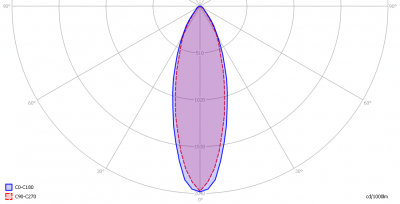The light diagram giving the radiation pattern.

It indicates the luminous intensity around the light bulb. The C0-C180 plane is along the length of the led bar, and the C90-C270 is perpendicular to that. The bundle is small (focused).

The unit is Cd/1000lm, meaning the intensity in Cd assuming there would be 1000 lumen in the measured light bulb. This enables comparing different types of light bulbs.

### Illuminance Ev at 1 m distance, or luminous intensity Iv

Herewith the plot of the averaged luminous intensity Iv as a function of the inclination angle with the light bulb.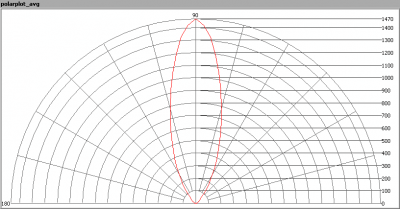The radiation pattern of the light bulb.

This radiation pattern is the average of the light output of the light diagram given earlier. Also, in this graph the luminous intensity is given in Cd.

These averaged values are used (later) to compute the lumen output.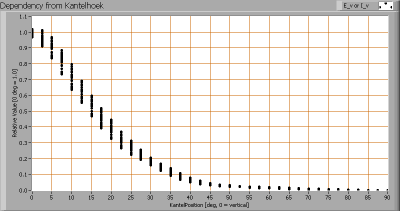Intensity data of every measured turn angle at each inclination angle.

This plot shows per inclination angle the intensity measurement results for each turn angle at that inclination angle. There are small differences in illuminance values for different turn angles (also seen in the light diagram, where there was little difference betwen C0-C180 and C70-C270). However for further calculations the averaged values will be used.

When using the average values per inclination angle, the beam angle can be computed, being 32 degrees.

### Luminous flux

With the averaged illuminance data at 1 m distance, taken from the graph showing the averaged radiation pattern, it is possible to compute the luminous flux.

The result of this computation for this light spot is a luminous flux of 727 lm.

### Luminous efficacy

The luminous flux being 727 lm, and the power of the lightbulb being 26.4 W, yields a luminous efficacy of 28 lm/W.

A power factor of 1 (since DC voltage is used) means that for every 1 kWh net power consumed, a reactive component of 0.0 kVAr was needed.

 Light bulb voltage 24 VDC Light bulb current 1.1 A Power P 26.4 W Apparent power S 26.4 VA Power factor 1.0

### Color temperature and Spectral power distribution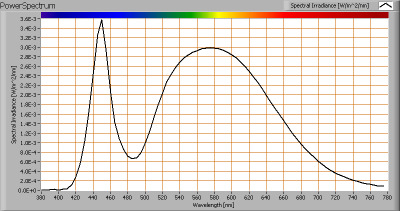The spectral power distribution of this light bulb.

The measured color temperature is about 4250 K which is neutral white.

This color temperature is measured straight underneath the light bulb. Below a graph showing the color temperature for different inclination angles.Color temperature as a function of inclination angle.

The color temperature is given for inclination angles up to 45 degrees, since at higher angles there is almost no light output anymore.

The value remains constant within 1.5 %.

### Chromaticity diagram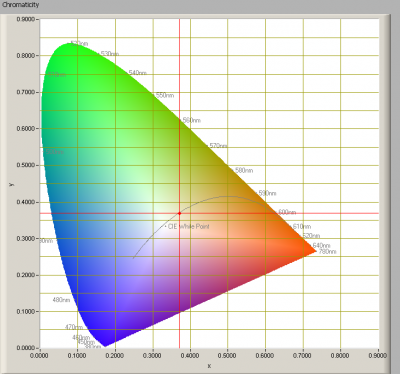The chromaticity space and the position of the lamp’s color coordinates in it.

The light coming from this lamp is on top of the Planckian Locus (the black path in the graph).

Its coordinates are x=0.3706 and y=0.3700.

### Color Rendering Index (CRI) or also Ra

Herewith the image showing the CRI as well as how well different colors are represented (rendered). The higher the number, the better the resemblance with the color when a black body radiator would have been used (the sun, or an incandescent lamp).

Each color has an index Rx, and the first 8 indexes (R1 .. R8) are averaged to compute the Ra which is equivalent to the CRI.CRI of the light of this lightbulb.

The value of 78 is a little bit lower than 80 which is considered a minimum value for indoor usage.

Note: the chromaticity difference is 0.0002 indicates the distance to the Planckian Locus. Its value is lower than 0.0054, which means that the calculated CRI result is meaningful.

### Voltage dependency

The dependency of a number of lamp parameters on the lamp voltage is determined. For this, the lamp voltage has been varied and its effect on the following lamp parameters measured: illuminance E_v [lx], the lamppower P [W] and the luminous efficacy [lm/W].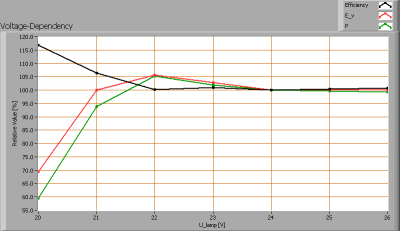Lamp voltage dependencies of certain light bulb parameters, where the value at 24 V is taken as 100 %.

The consumed power and the illuminance vary insignificantly when the voltage varies between 21-26 V. It drops sharply below the 21 V.

When the voltage at 24 V varies with + and – 1 V, then the illuminance varies 2.5 %, so when abrupt voltage changes occur this effect is not visible in the illuminance output.

### Warm up effects

After switch on of a cold lamp, the effect of heating up of the lamp is measured on illuminance E_v [lx], color temperature CT or correlated color temperature CCT [K], the lamppower P [W] and the luminous efficacy [lm/W].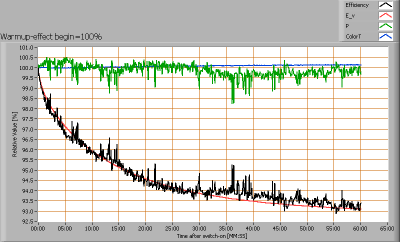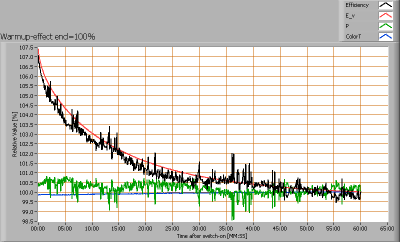Effect of warming up on different light bulb parameters. At top the 100 % level is put at begin, and at bottom at the end.

Note that the voltage unit, converting the mains voltage from 230 V AC into 24 V DC, is included in this measurement. The readings for the illumination are significant and come from the led bar only. The readings for the power are the sum of the voltage unit and the led bar, so these values need not be considered.

The illumination decreases with only 7 %, which is little.